# Value Nodes

The Value Nodes group includes nodes that generate or modify images of color mode VALUE.

Images of color mode VALUE store a 32 bit floating point value on each pixel. The image header knows the minimum and maximum value on the image, and renders the image in the viewport as a monochromatic image, where the minimum value is represented by black, and the maximum value is represented by white.

The main advantage of the VALUE color mode is that images can represent the height 3D elements, and they can be mixed with other images using nodes like Add or Multiply in a more natural way.

•Value nodes

•To Value node

•Value Add node

•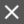Value Multiply node

•Value Minimum node

•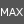Value Maximum node

•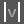Value Absolute node

•Value Condition node

•Value Clamp node

•Value Steps node

•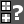Value Compare node

•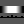Value Range node

•Value Function node

•Relief node

•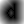Distance node

•[Compute Gradient node](NodesValueCompute Gradient.html)

Main Page